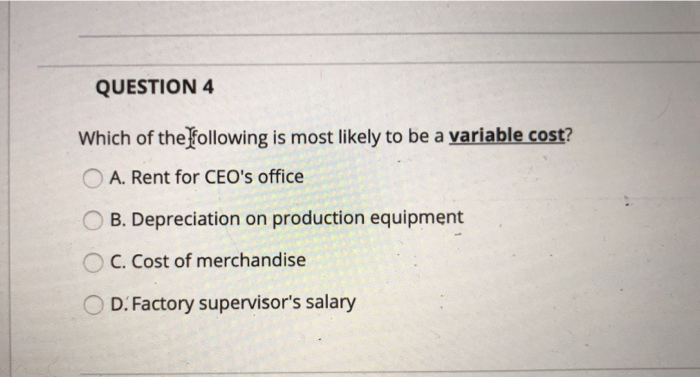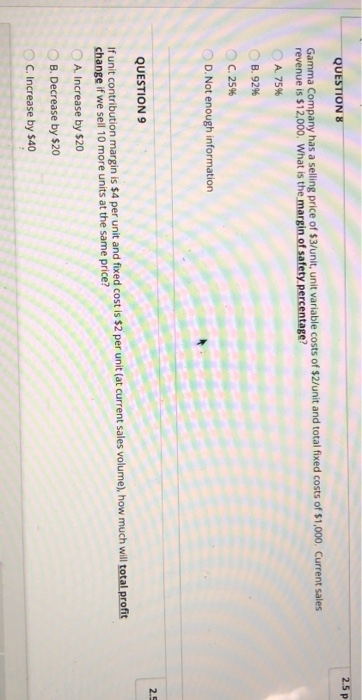### Create an Account

Home / Questions / which of the following is most likely to be variable cost QUESTION 4 Which of the following is most

# which of the following is most likely to be variable cost QUESTION 4 Which of the following is most

which of the following is most likely to be variable costQUESTION 4 Which of the following is most likely to be a variable cost? O A. Rent for CEO&#39;s office OB. Depreciation on production equipment O C. Cost of merchandise O D. Factory supervisor&#39;s salary 2.5 p QUESTION 8 Gamma Company has a selling price of \$3/unit, unit variable costs of \$2/unit and total fixed costs of \$1,000. Current sales revenue is \$12,000. What is the margin of safety percentage? A 75% B. 92% C.25% D. Not enough information 2.5 QUESTION 9 If unit contribution margin is \$4 per unit and fixed cost is \$2 per unit (at current sales volume), how much will total profit change if we sell 10 more units at the same price? A. Increase by \$20 B. Decrease by \$20 C. Increase by \$40

Apr 03 2020 View more View LessSubscribe To Get Solution